gemseo / datasets

# io_dataset module¶

A Dataset to store input and output values.

class gemseo.datasets.io_dataset.IODataset(data=None, index=None, columns=None, dtype=None, copy=None, *, dataset_name='')[source]

Bases: Dataset

A Dataset to store input and output values.

Warning

A Dataset behaves like any multi-index DataFrame but its instantiation using the constructor dataset = Dataset(data, ...) can lead to some inconsistencies (multi-index levels, index values, dtypes, …). Hence, the construction from the dedicated methods is recommended, e.g. dataset = Dataset(); dataset.add_variable("x", data).

Notes

The columns of a data structure (NumPy array, DataFrame, Dataset, …) are called features. The features of a Dataset include all the components of all the variables of all the groups.

Initialize self. See help(type(self)) for accurate signature.

Parameters:
• dataset_name (str) –

The name of the dataset.

By default it is set to “”.

• data (ndarray | Iterable | dict | DataFrame | None) –

• index (Axes | None) –

• columns (Axes | None) –

• dtype (Dtype | None) –

• copy (bool | None) –

Add the data related to the input group.

Parameters:
• data (DataType) – The data.

• variable_names (StrColumnType) –

The names of the variables. If empty, use DEFAULT_VARIABLE_NAME.

By default it is set to ().

• variable_names_to_n_components (dict[str, int] | None) – The number of components of the variables. If variable_names is empty, this argument is not considered. If None, assume that all the variables have a single component.

Return type:

None

Add data related to an input variable.

Parameters:
• variable_name (str) – The name of the variable.

• data (ndarray | Iterable[Any] | Any) – The data, either an array shaped as (n_entries, n_features), an array shaped as (n_entries,) that will be reshaped as (n_entries, 1) or a scalar that will be converted into an array shaped as (n_entries, 1).

• components (int | Iterable[int]) –

The components considered. If empty, use [0, ..., n_features].

By default it is set to ().

Return type:

None

Add the data related to the output group.

Parameters:
• data (DataType) – The data.

• variable_names (StrColumnType) –

The names of the variables. If empty, use DEFAULT_VARIABLE_NAME.

By default it is set to ().

• variable_names_to_n_components (dict[str, int] | None) – The number of components of the variables. If variable_names is empty, this argument is not considered. If None, assume that all the variables have a single component.

Return type:

None

Add data related to an output variable.

Parameters:
• variable_name (str) – The name of the variable.

• data (ndarray | Iterable[Any] | Any) – The data, either an array shaped as (n_entries, n_features), an array shaped as (n_entries,) that will be reshaped as (n_entries, 1) or a scalar that will be converted into an array shaped as (n_entries, 1).

• components (int | Iterable[int]) –

The components considered. If empty, use [0, ..., n_features].

By default it is set to ().

Return type:

None

INPUT_GROUP: Final[str] = 'inputs'

The group name for the input variables.

OUTPUT_GROUP: Final[str] = 'outputs'

The group name for the output variables.

property input_dataset: IODataset

The view of the input dataset.

property input_names: list[str]

The names of the inputs.

misc: dict[str, Any]

Miscellaneous information specific to the dataset, and not to an entry.

property n_samples: int

The number of samples.

name: str

The name of the dataset.

property output_dataset: IODataset

The view of the output dataset.

property output_names: list[str]

The names of the outputs.

property samples: list[int | str]

The ordered samples.

## Examples using IODataset¶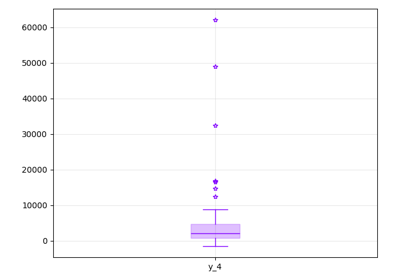Empirical estimation of statistics

Empirical estimation of statistics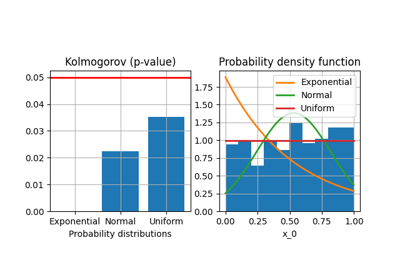Parametric estimation of statistics

Parametric estimation of statistics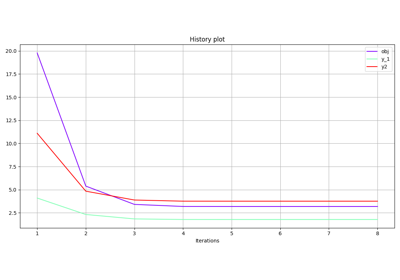Store observables

Store observables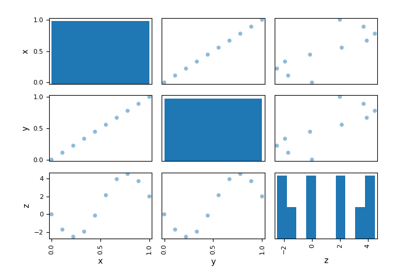Diagonal design of experiments

Diagonal design of experiments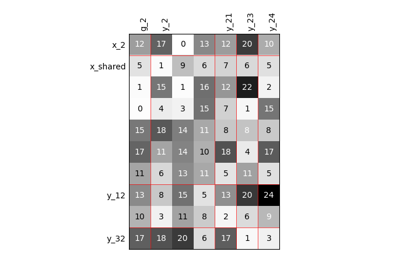Scalable diagonal discipline

Scalable diagonal disciplineUse a design of experiments from a file

Use a design of experiments from a fileUse a design of experiments from an array

Use a design of experiments from an arrayCreate a surrogate discipline

Create a surrogate disciplinePlug a surrogate discipline in a Scenario

Plug a surrogate discipline in a Scenario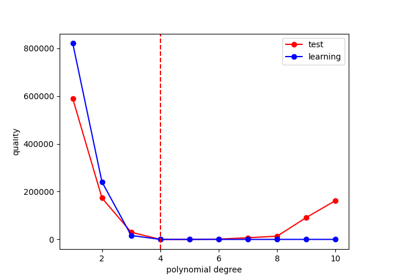Calibration of a polynomial regression

Calibration of a polynomial regression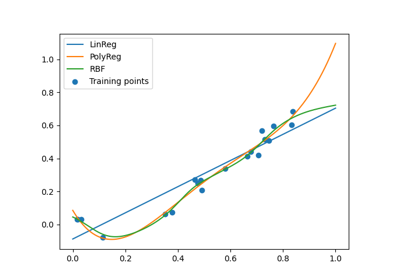Machine learning algorithm selection example

Machine learning algorithm selection exampleClassification API

Classification APIK nearest neighbors classification

K nearest neighbors classificationRandom forest classification

Random forest classificationAPI

API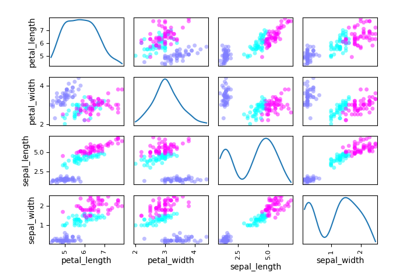Gaussian Mixtures

Gaussian Mixtures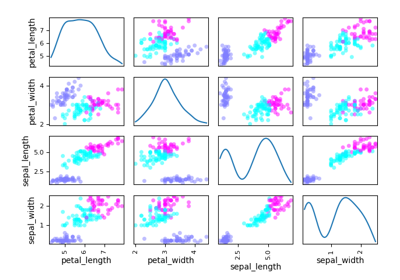K-means

K-means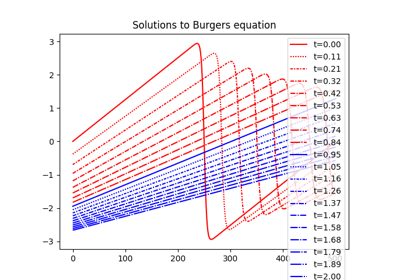KL-SVD on Burgers equation

KL-SVD on Burgers equation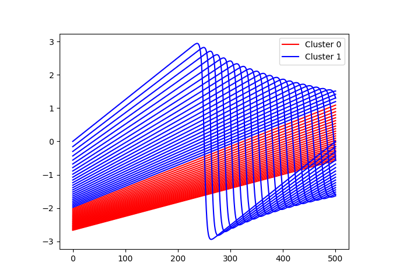Mixture of experts with PCA on Burgers dataset

Mixture of experts with PCA on Burgers dataset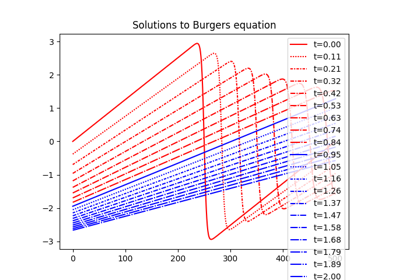PCA on Burgers equation

PCA on Burgers equation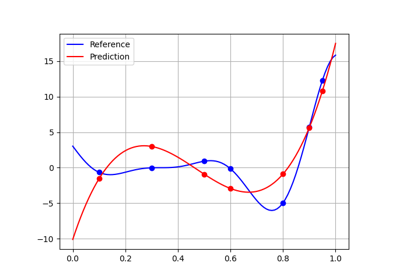MSE example - test-train split

MSE example - test-train splitQuality measure for surrogate model comparison

Quality measure for surrogate model comparisonAPI

API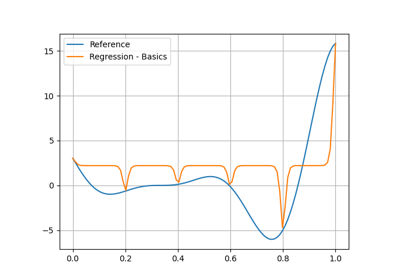GP regression

GP regression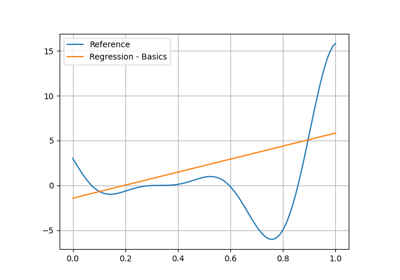Linear regression

Linear regression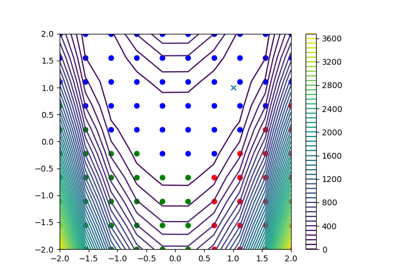Mixture of experts

Mixture of experts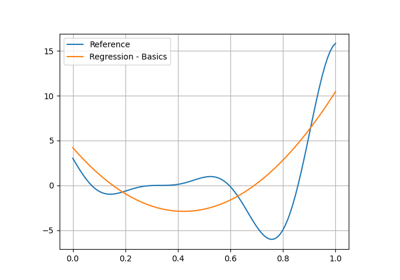PCE regression

PCE regression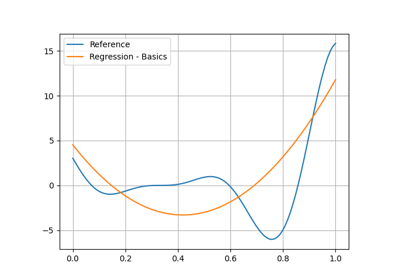Polynomial regression

Polynomial regression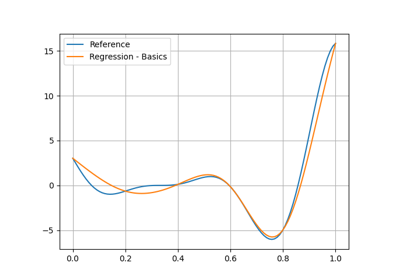RBF regression

RBF regression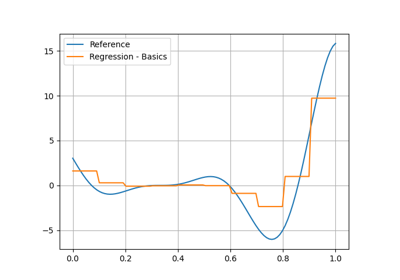Random forest regression

Random forest regressionThe input-output dataset

The input-output dataset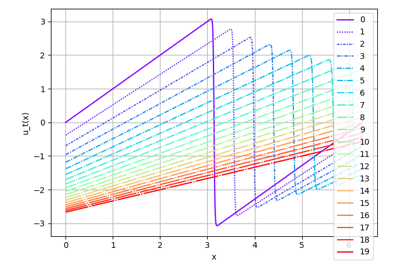Burgers dataset

Burgers dataset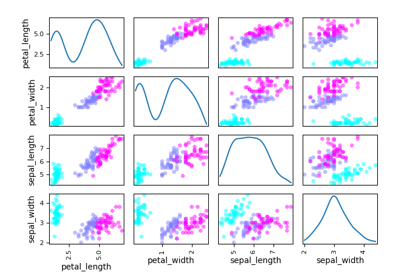Iris dataset

Iris dataset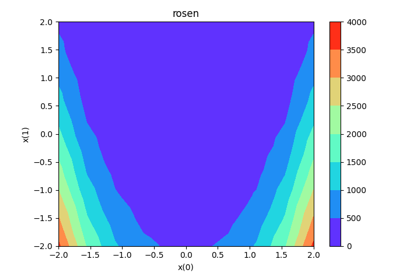Rosenbrock dataset

Rosenbrock dataset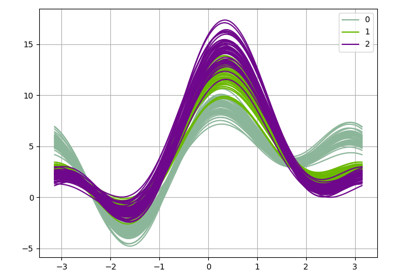Andrews curves

Andrews curves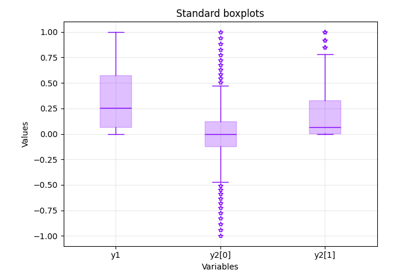Boxplot

Boxplot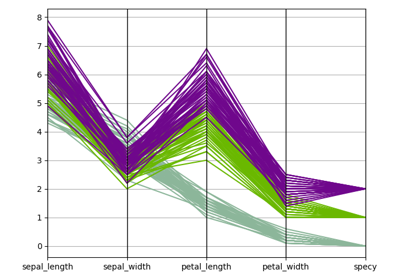Parallel coordinates

Parallel coordinates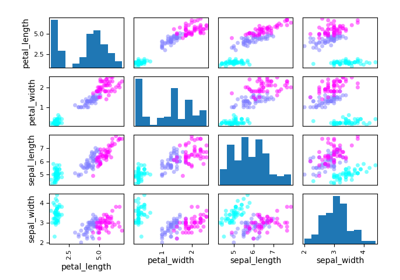Scatter matrix

Scatter matrix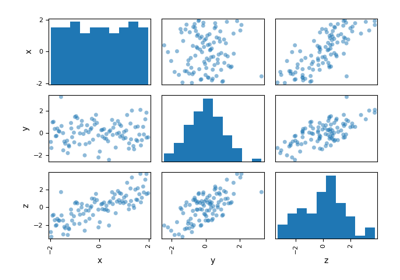Parameter space

Parameter space